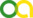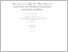Süß, Daniel (2018). Due to, or in spite of? The effect of constraints on efficiency in quantum estimation problems. PhD thesis, Universität zu Köln.Preview
PDF
thesis.pdf - Accepted Version

## Abstract

In this thesis, we study the interplay of constraints and complexity in quantum estimation. We investigate three inference problems, where additional structure in the form of constraints is exploited to reduce the sample and/or computational complexity. The first example is concerned with uncertainty quantification in quantum state estimation, where the positive-semidefinite constraint is used to construct more powerful, that is smaller, error regions. However, as we show in this work, doing so in an optimal way constitutes a computationally hard problem, and therefore, is intractable for larger systems. The second inference problem deals with phase retrieval and its application to characterizing linear optical circuits. The main chal- lenge here is the fact that the measurements are insensitive to complex phases, and hence, reconstruction requires deliberate utilization of interference. We propose a reconstruction algorithm based on ideas from low-rank matrix recovery. More specifically, we map the problem of reconstruction from phase-insensitive measurements to the problem of recovering a rank-one matrix from linear measurements. For the efficient solution of the latter it is crucial to exploit the rank-one constraint. Finally, we investigate low-rank tensor recovery – the problem of reconstructing a low-complexity tensor embedded in an exponentially large space. We provide evidence that with high probability succesful recovery is feasible by Gaussian product tensors with the number of measurements only depending on the target’s intrinsic complexity, and hence, scaling polynomially in the order of tensor. Therefore, the low-rank constraint can be exploited to dramatically reduce the sample complexity of the problem. Additionally, the use of measurement tensors with an efficient representation is necessary for computational efficiency.

Item Type: Thesis (PhD thesis)
Creators:
CreatorsEmailORCIDORCID Put Code
Süß, Danielsuess.daniel@gmail.comUNSPECIFIEDUNSPECIFIED
URN: urn:nbn:de:hbz:38-90128
Date: 13 November 2018
Language: English
Faculty: Faculty of Mathematics and Natural Sciences
Divisions: Faculty of Mathematics and Natural Sciences > Department of Physics > Institute for Theoretical Physics
Subjects: Mathematics
Physics
Uncontrolled Keywords:
KeywordsLanguage
quantum estimationEnglish
complexityEnglish
Date of oral exam: 13 September 2018
Referee:
Gross, DavidProf. Dr.
Berg, JohannesProf. Dr.
Refereed: Yes
URI: http://kups.ub.uni-koeln.de/id/eprint/9012View Item